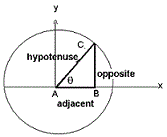### Trigonometry Calculator

 The trigonometric functions have been defined using the unit circle. The degree of an angle measured counter-clock-wise from the x-axis along an arc of the circle. The sin(θ) is the vertical component , the cos(θ) is the horizontal coordinate of the arc endpoint and the ratio of sin(θ) / cos(θ) is defined as tan(θ). Since the trigonometric functions are periodic with period 360 degree or say 2 π therefore : func(θ) = func( 2 π r n + θ) where func is a trigonometric function and n is an integer. Sin(q) = Opposite / Hypotenuse Cos(q) = Adjacent / Hypotenuse Tan(q) = Opposite / AdjacentPlease enter value:
Radians
 Sine(Sin) Cosine(Cos) Tangent(Tan) Cotangent(Cot) Secant(Sec) Cosecant(Cosec)

 Arc Sine(ASin) Arc Cosine(ACos) Arc Tangent(ATan) Arc Cotangent(ACot) Arc Secant(ASec) Arc Cosecant(ACosec)

Degrees
 Sine(Sin) Cosine(Cos) Tangent(Tan) Cotangent(Cot) Secant(Sec) Cosecant(Cosec)

 Arc Sine(ASin) Arc Cosine(ACos) Arc Tangent(ATan) Arc Cotangent(ACot) Arc Secant(ASec) Arc Cosecant(ACosec)

The table for basic trigonometric numbers of the most common angles as follows:
 Angle sin cos tan cot sec cosec 0o 0 1 0 Not define 1 Not define 30o 1/2 √3/2 1/√3 √3 2/√3 2 45o 1/√2 1/√2 1 1 √2 √2 60o √3/2 1/2 √3 1/√3 2 2/√3 90o 1 0 Not define 0 Not define 1